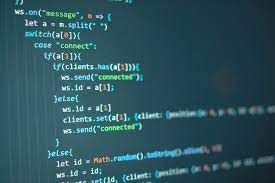# How to Use Variable As Key in JavaScript Object

JavaScript objects allow you to store different types of data in a compact manner as key-value pairs. They allow you to directly access these values using keys, without the need for any iteration. Generally, the key values are literal strings. But sometimes you may need to use variable as key in JavaScript object. In this article, we will learn how to do this.

## How to Use Variable As Key in JavaScript Object

Here is an example of a JavaScript object.

`var d = {'id':123, 'name':'john'};`

As you can see, the keys id and name are literal strings. Let us say you have the following variable key2 and want to use it in place of ‘name’ key above.

`var key2 = 'name';`

Typically developers use the following method to use variable as key in JS object.

`var d = {'id':123, key2:'john'};`

But if you try to use the key this way, then when you try to access the value of object with variable

`console.log(d[key2]);`

or if you try accessing the value using ‘name’ literal string

`console.log(d['name'])`

you will get the value as undefined. On the other hand, if you try to use variable name as literal string.

`console.log(d['key2']);`

you will get the following value.

`john`

This is because JavaScript treats the variable name as a literal string and not a variable. To overcome this problem, you need to define an empty object first and then assign its value using a variable as a key.

```e ={};
e[key2]='jim';
console.log(e[key2]);```

When you run the above code, you will get the following value.

`jim`

Here is another way to use variable as key in JS object. In this method, you need to enclose the variable in square brackets [] as shown below.

```var key2 = 'name';
var e ={[key2]:'jim'};
console.log(e[key2]);```

To summarize, the following way of using variable as key DOES NOT WORK.

```var key2 = 'name';
var d = {'id':123, key2:'john'};```

But the following ways of using variable as key WORKS.

```//approach 1
var key2 = 'name';
var e ={};
e[key2]='jim';
console.log(e[key2]);

//aproach 2
var key2 = 'name';
var e ={[key2]:'jim'};
console.log(e[key2]);```

In this article, we have learnt a couple of ways to use variable as key in JavaScript object. You can use either of these methods as per your requirement. Either you can create an empty object and then use variable as key to store value, or you can enclose variable in square brackets, if you want to use variable while defining the object itself.